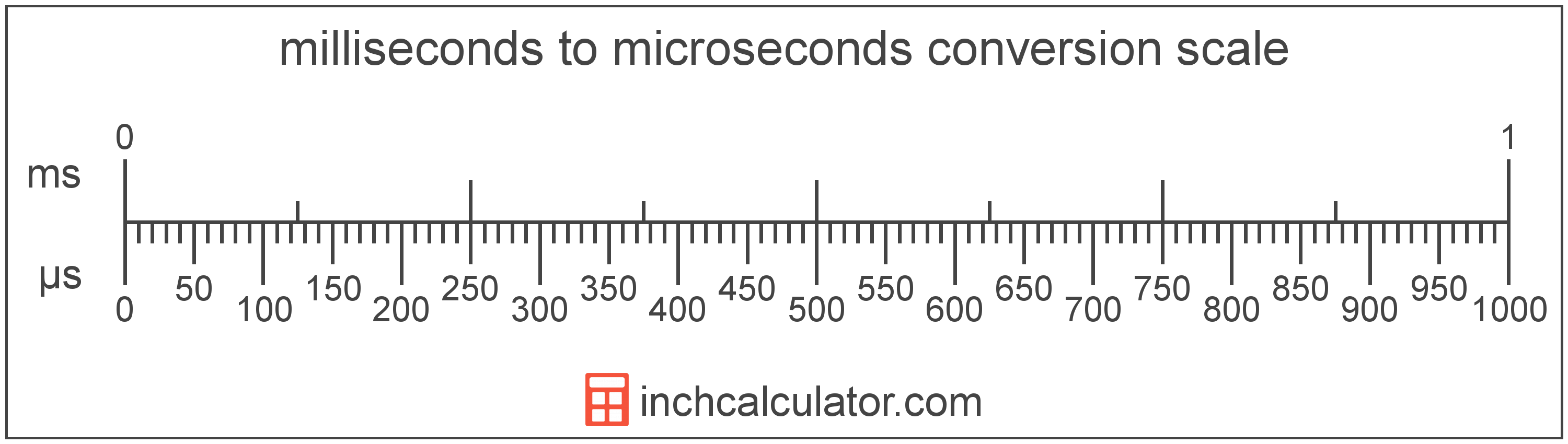# Microseconds to Milliseconds Converter

Enter the time in microseconds below to get the value converted to milliseconds.

Results in Milliseconds:1 µs = 0.001 ms

Do you want to convert milliseconds to microseconds?

## How to Convert Microseconds to Milliseconds

To convert a measurement in microseconds to a measurement in milliseconds, divide the time by the following conversion ratio: 1,000 microseconds/millisecond.

Since one millisecond is equal to 1,000 microseconds, you can use this simple formula to convert:

milliseconds = microseconds ÷ 1,000

The time in milliseconds is equal to the time in microseconds divided by 1,000.

For example, here's how to convert 5,000 microseconds to milliseconds using the formula above.
milliseconds = (5,000 µs ÷ 1,000) = 5 ms## What Is a Microsecond?

One microsecond is equal to 1/1,000 of a millisecond or 1,000 nanoseconds.

The microsecond is a multiple of the second, which is the SI base unit for time. In the metric system, "micro" is the prefix for millionths, or 10-6. Microseconds can be abbreviated as µs; for example, 1 microsecond can be written as 1 µs.

## What Is a Millisecond?

One millisecond is equal to 1/1,000 of a second or 1,000 microseconds.

The millisecond is a multiple of the second, which is the SI base unit for time. In the metric system, "milli" is the prefix for thousandths, or 10-3. Milliseconds can be abbreviated as ms; for example, 1 millisecond can be written as 1 ms.

## Microsecond to Millisecond Conversion Table

Table showing various microsecond measurements converted to milliseconds.
Microseconds Milliseconds
1 µs 0.001 ms
2 µs 0.002 ms
3 µs 0.003 ms
4 µs 0.004 ms
5 µs 0.005 ms
6 µs 0.006 ms
7 µs 0.007 ms
8 µs 0.008 ms
9 µs 0.009 ms
10 µs 0.01 ms
20 µs 0.02 ms
30 µs 0.03 ms
40 µs 0.04 ms
50 µs 0.05 ms
60 µs 0.06 ms
70 µs 0.07 ms
80 µs 0.08 ms
90 µs 0.09 ms
100 µs 0.1 ms
200 µs 0.2 ms
300 µs 0.3 ms
400 µs 0.4 ms
500 µs 0.5 ms
600 µs 0.6 ms
700 µs 0.7 ms
800 µs 0.8 ms
900 µs 0.9 ms
1,000 µs 1 ms